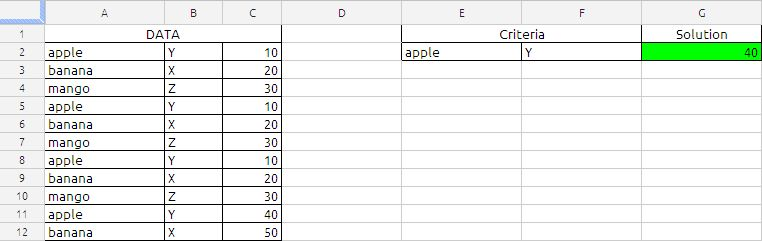# Maximum Based on Multiple CriteriaThe Formula in cell G2 is
=ArrayFormula(MAX(IF(A2:A12=E2,IF(B2:B12=F2,C2:C12))))

the above formula will return the maximum value in cell C2:C12 corresponding to criteria specified in the cell E2 and F2.

the formula will narrows down using the conditions in the IF function first A2:A12 and then B2:B12. If the both conditions are met the resultant will be value in cell C2:C12

Which will be MAX {10,10,10,40}

based on the sample data the result will be 40.

Advertisements

### One Response to Maximum Based on Multiple Criteria

1.Rajesh Mehta says:

Please help in your below formula, how can it be “continue” in all rows automatically?

=ArrayFormula(MAX(IF(A2:A12=E2,IF(B2:B12=F2,C2:C12))))